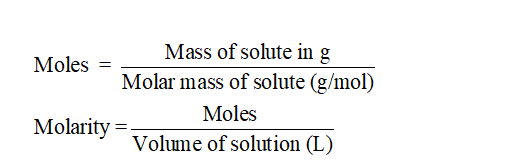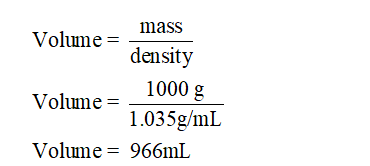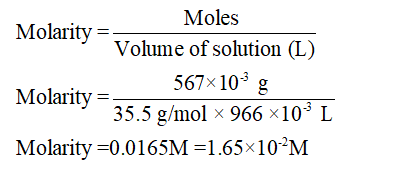# A sample of seawater from a tidal estuary was found to contain a concentration of 567 mg of chloride ion per kg of seawater. If the density of the sample was 1.035 g/mL, what is the molarity of the chloride ion?

Question
12 views

A sample of seawater from a tidal estuary was found to contain a concentration of 567 mg of chloride ion per kg of seawater. If the density of the sample was 1.035 g/mL, what is the molarity of the chloride ion?

check_circle

Step 1

Solution stoichiometry is mainly based on the calculation of moles and volumes. These two values are used to calculate the molarity of solution. The relation between moles, and mass is as given below;Step 2

Given:

Density = 1.035 g/mL

Mass of sea water = 1 kg = 1000 g

Calculate volume of sea water:Step 3

Mass of Chloride ions = 567 mg = 567 x 10-3 g

Molar Mass of Chloride ions = 35.5 g/mol

Calculate molarity:...

### Want to see the full answer?

See Solution

#### Want to see this answer and more?

Solutions are written by subject experts who are available 24/7. Questions are typically answered within 1 hour.*

See Solution
*Response times may vary by subject and question.
Tagged in